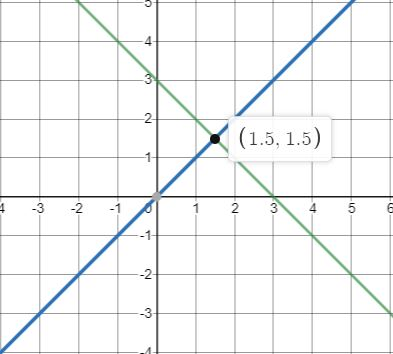New update is available. Click here to update.

# Ninja And Geometry

Last Updated: 25 Feb, 2021
Difficulty: Easy

## PROBLEM STATEMENT

#### Note:

``````1. You do not need to fix the output up to 6 decimal places. it has already been taken care of. Just return the output in the data type mentioned in the function.
2. Lines ‘AB’ and ‘PQ’ are two different lines.
``````

#### For Example: For A(0, 3), B(3, 0) and P(0, 0), Q(5, 5) the point of intersection of line ‘AB’ and ‘PQ’ is (1.500000, 1.500000). As shown below:#### As Ninja is weak in Geometry, can you help him to find the intersection of these two lines?

##### Input Format
``````The first line of input contains an integer ’T’ which denotes the number of test cases or queries to be run. Then the test cases follow.

The first line of each test case contains 8 single space-separated integers ‘AX1’,’ AY1’, ’BX2’, ’BY2’, ’PX1’, ’PY1’, ’QX2’, ’QY2’ where ’AX1’ represents the ‘X’ coordinate of the point ‘A’ and ‘AY1’ represents the ‘Y’ coordinate of the point ‘A’ and so on.
``````
##### Output Format :
``````For each test case, print the ‘X’ and ‘Y’ coordinates of the point of intersection of the two lines ‘AB’  and ‘PQ’ up to 6 decimal places.

Print the output of each test case in a separate line.
``````

#### Note:

``````You do not need to print anything; it has already been taken care of. Just implement the given function.
``````
##### Constraints:
``````1 <= ‘T’ <= 10^2
1 <= ‘N’ <= 5*10^3
1 <= ‘AX1’, ’AY1’, ’BX2’, ’BY2’, ’PX1’, ’PY1’, ’QX2’, ’QY2’ <= 10^5

Where ‘T’ denotes the total number of test cases, ‘N’ represents the number of boxes, ‘AX1’, ’AY1’, ’BX2’, ’BY2’, ’PX1’, ’PY1’, ’QX2’, ’QY2’ represents the the ‘X’ coordinate of the point ‘A’ and ‘AY1’ represents the ‘Y’ coordinate of the point ‘A’ and so on.

Time Limit: 1 sec
``````## Approach 1

The idea behind this approach is to derive the equation of both of the lines ‘AB’ and ‘PQ’ and check if the Slope of these two lines is equal or not. Following are the two cases:

• If the slope of ‘AB’ and ‘PQ’ is the same that means they are parallel so we return -1.
• Else there must a point where ‘AB’ and ‘PQ’ are intersecting.

For a better understanding of this approach, Assume that we have two points which are A(a1, b1) and B(a2, b2). Now, we have to find the equation of line formed by points ‘A’ and ‘B’.

• The equation of a line in geometry is :
• a1(X)  +  b1(Y) = c1 (equation 1)
• a2(X)  +  b2(Y) = c2 (equation 2)

Now we have to solve these 2 equations to get the point of intersection of these two lines. To solve this problem, we can multiply equation 1 by b2 and equation 2 by b1,this gives us the following result:

• a1b2(X)  +  b1b2(Y) = c1b2 (equation 1)
• a2b1(X)  +  b2b1(Y) = c2b1 (equation 2)
• Subtracting equation 2 from equation 1 we get the following result:
• (a1b2  –  a2b1) (X)  =  c1b2  –  c2b1 (equation 3)
• This can give us the value of X. Similarly, we can find out the value of the Y.So (X, Y) is the point of intersection of these two lines.
• If the coefficient of X in equation 3 is 0 that means lines the parallel. because in that case the equation becomes invalid and  (a1b2  –  a2b1) represents the difference between the slopes of the two lines.
• slopeDiff’ = a1 b2 - a2 b1

Here is the complete algorithm:

1. Find the difference between the slopes of the two lines i.e. ‘slopeDiff’ = ‘a1’ * ‘ b2’  -  ‘a2’ * ‘ b1
2. If ‘slopeDiff’ is 0 that means lines are parallel. Return -1.
3. Else following are the two cases:
• The ‘X’ coordinate of the point of the intersection is  (‘c1’ * ‘b2’ -  ‘c2’ * ‘b1’) / ‘slopeDiff’.
• The ‘Y’ coordinate of the point of the intersection is  (‘a1’ * ‘c2’ -  ‘a2’ * ‘c1’) / ‘slopeDiff
4. Finally, insert these coordinates into an array/list and return the array/list.